# Dihedron

Set of regular n-gonal dihedra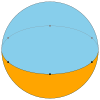Example hexagonal dihedron on a sphere
Type Regular polyhedron or spherical tiling
Faces 2 n-gons
Edges n
Vertices n
Vertex configuration n.n
Wythoff symbol 2 | n 2
Schläfli symbol {n,2}
Coxeter diagramSymmetry group Dnh, [2,n], (*22n), order 4n
Rotation group Dn, [2,n]+, (22n), order 2n
Dual polyhedron hosohedron

A dihedron is a type of polyhedron, made of two polygon faces which share the same set of edges. In three-dimensional Euclidean space, it is degenerate if its faces are flat, while in three-dimensional spherical space, a dihedron with flat faces can be thought of as a lens, an example of which is the fundamental domain of a lens space L(p,q).

Usually a regular dihedron is implied (two regular polygons) and this gives it a Schläfli symbol as {n,2}. Each polygon fills a hemisphere, with a regular n-gon on a great circle equator between them.

The dual of a n-gonal dihedron is the n-gonal hosohedron, where n digon faces share two vertices.

## As a polyhedron

A dihedron can be considered a degenerate prism consisting of two (planar) n-sided polygons connected "back-to-back", so that the resulting object has no depth.

## As a tiling on a sphere

As a spherical tiling, a dihedron can exist as nondegenerate form, with two n-sided faces covering the sphere, each face being a hemisphere, and vertices around a great circle. (It is regular if the vertices are equally spaced.)

The regular polyhedron {2,2} is self-dual, and is both a hosohedron and a dihedron.

 Schläfli Coxeter Image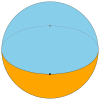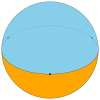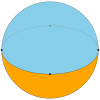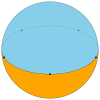{2,2} {3,2} {4,2} {5,2} {6,2}...Faces 2 {2} 2 {3} 2 {4} 2 {5} 2 {6} Edges andvertices 2 3 4 5 6

## Apeirogonal dihedron

In the limit the dihedron becomes an apeirogonal dihedron as a 2-dimensional tessellation:## Ditopes

A regular ditope is an n-dimensional analogue of a dihedron, with Schläfli symbol {p, ... q,r,2}. It has two facets, {p, ... q,r}, which share all ridges, {p, ... q} in common.

1. Gausmann, Evelise; Roland Lehoucq; Jean-Pierre Luminet; Jean-Philippe Uzan; Jeffrey Weeks (2001). "Topological Lensing in Spherical Spaces". Classical and Quantum Gravity. 18: 5155–5186. arXiv:gr-qc/0106033. doi:10.1088/0264-9381/18/23/311.